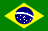# JavaScript: Compound Gain Calculator

This program calculates the daily, monthly and annual gain for an account where interest accrues daily and where you always leave yesterday's inter­est in the account so it becomes part of today's capital that generates to­day's interest.

Annual Daily Monthly Percentages Multipliers

Enter a sensible percentage or a multiplier into one of the fields and then click the mouse somewhere in the coloured area (not in an entry field). The program should then calculate and display the corresponding figures for the other fields. As well as being useful for compound interest, this calculator should also serve well as a rough guide when trying to correlate the different ways people quote share perfor­mance. However, shares do not gain (or lose) the same amount every day over a year, so the program can calculate only a mean daily gain from an annual or monthly figure.

By default, the program assumes that gain is accrued for every day of every month of the year. However, if you are working with generators that only produce gain on trading days, then you need to enter the number of actual trading days in a month and a year, which I think is usually 261 per year and 21 per month.

To compute monthly gain from a quoted annual gain, the program calculates the 365th root of the annual inflation multiplier and then raises this to the 30th power. To compute annual gain from a quoted monthly gain, the program calculates the 30th root of the monthly inflation multiplier and then raises this to the 365th power. Of course these roots and powers will be different if you enter different numbers for trading days in the year and the month.© January 2004 Robert John Morton Clique na bandeira para ler em Português.